• Problem Description 设DFA M=({S,U,V,Q},{a,b},f, S, {Q}) 其中f定义为:...编写一个DFA程序，用于判断符号串t是否被DFA M接受？ Input 输入多行∑*上的符号串t，输入EOF结束。 Output 判断每行的符号串t能够被DFA M识.

Problem Description
设DFA M=({S,U,V,Q},{a,b},f, S, {Q}) 其中f定义为: f(S,a)=U f(V,a)=U f(S,b)=V f(V,b)=Q f(U,a)=Q f(Q,a)=Q f(U,b)=V f(Q,b)=Q DFA的状态转换关系如所示。编写一个DFA程序，用于判断符号串t是否被DFA M接受？ Input 输入多行∑*上的符号串t，输入EOF结束。 Output 判断每行的符号串t能够被DFA M识别，如果可以识别，输出"accept"；否则，输出"not accept"。

Sample Input baab ab Sample Output accept not accept

代码：C++实现
#include <iostream>

using namespace std;

char f(char v1, char v2) {
char nextState;
if (v2 == 'a') {
if (v1 == 'S') {   nextState = 'U';		}
else if (v1 == 'U'){		nextState = 'Q';		}
else if (v1 == 'V'){		nextState = 'U';		}
else if (v1 == 'Q'){		nextState = 'Q';		}
}
else if (v2 =='b'){
if (v1 == 'S') {			nextState = 'V';		}
else if (v1 == 'U'){		nextState = 'V';		}
else if (v1 == 'V'){		nextState = 'Q';		}
else if (v1 == 'Q'){		nextState = 'Q';		}
}
return nextState;
}

int DFA(char* s) {
char S = 'S';
for (int i = 0; s[i]!='\0'; i++) {
S = f(S, s[i]);
}
if (S == 'Q') {
return 1;
}
else {
return 0;
}
}

int main() {
char s;
while(cin>>s){
if(DFA(s)) cout << "accept" << endl;
if(DFA(s)==0)cout << "not accept" << endl;
}
return 0;
}

展开全文c++ 编译器
• 令s是一个代表状态，而且假设：在DFA M中，输入为a时从s到t转换。令t所在组的代表是r,那么在M’中有一个从s到r的转换，标记为a。令包含s0的状态组的代表是M’的开始状态，并令M’的接受状态是那些属于F的状态所在...
• 敏感词过滤算法DFA-确定有穷自动机 DFA全称为：Deterministic Finite Automaton , 即确定有穷自动机。其特征为：有一个有限状态集合和一些从一个状态通向另一个状态的边，每条边上标记有一个符号，其中一个状态是...
敏感词过滤算法DFA-确定有穷自动机
DFA全称为：Deterministic Finite Automaton , 即确定有穷自动机。其特征为：有一个有限状态集合和一些从一个状态通向另一个状态的边，每条边上标记有一个符号，其中一个状态是初态，某些状态是终态。但不同于不确定的有限自动机，DFA中不会有从同一状态出发的两条边标志有相同的符号。
检索的过程，就是hashMap的get实现

1、第一个字“冰”，我们在hashMap中可以找到。得到一个新的map = hashMap.get("")。
2、如果map == null，则不是敏感词。否则跳至3
3、获取map中的isEnd，通过isEnd是否等于1来判断该词是否为最后一个。如果isEnd == 1表示该词为敏感词，否则跳至1。
通过这个步骤我们可以判断“冰毒”为敏感词，但是如果我们输入“冰箱”则不是敏感词了。

使用我编写好的工具类，更加方便

import java.util.*;

public class SensitiveWordUtil {

public static Map<String, Object> dictionaryMap = new HashMap<>();

/**
* 生成关键词字典库
* @param words
* @return
*/
public static void initMap(Collection<String> words) {
if (words == null) {
System.out.println("敏感词列表不能为空");
return ;
}

// map初始长度words.size()，整个字典库的入口字数(小于words.size()，因为不同的词可能会有相同的首字)
Map<String, Object> map = new HashMap<>(words.size());
// 遍历过程中当前层次的数据
Map<String, Object> curMap = null;
Iterator<String> iterator = words.iterator();

while (iterator.hasNext()) {
String word = iterator.next();
curMap = map;
int len = word.length();
for (int i =0; i < len; i++) {
// 遍历每个词的字
String key = String.valueOf(word.charAt(i));
// 当前字在当前层是否存在, 不存在则新建, 当前层数据指向下一个节点, 继续判断是否存在数据
Map<String, Object> wordMap = (Map<String, Object>) curMap.get(key);
if (wordMap == null) {
// 每个节点存在两个数据: 下一个节点和isEnd(是否结束标志)
wordMap = new HashMap<>(2);
wordMap.put("isEnd", "0");
curMap.put(key, wordMap);
}
curMap = wordMap;
// 如果当前字是词的最后一个字，则将isEnd标志置1
if (i == len -1) {
curMap.put("isEnd", "1");
}
}
}

dictionaryMap = map;
}

/**
* 搜索文本中某个文字是否匹配关键词
* @param text
* @param beginIndex
* @return
*/
private static int checkWord(String text, int beginIndex) {
if (dictionaryMap == null) {
throw new RuntimeException("字典不能为空");
}
boolean isEnd = false;
int wordLength = 0;
Map<String, Object> curMap = dictionaryMap;
int len = text.length();
// 从文本的第beginIndex开始匹配
for (int i = beginIndex; i < len; i++) {
String key = String.valueOf(text.charAt(i));
// 获取当前key的下一个节点
curMap = (Map<String, Object>) curMap.get(key);
if (curMap == null) {
break;
} else {
wordLength ++;
if ("1".equals(curMap.get("isEnd"))) {
isEnd = true;
}
}
}
if (!isEnd) {
wordLength = 0;
}
return wordLength;
}

/**
* 获取匹配的关键词和命中次数
* @param text
* @return
*/
public static Map<String, Integer> matchWords(String text) {
Map<String, Integer> wordMap = new HashMap<>();
int len = text.length();
for (int i = 0; i < len; i++) {
int wordLength = checkWord(text, i);
if (wordLength > 0) {
String word = text.substring(i, i + wordLength);
// 添加关键词匹配次数
if (wordMap.containsKey(word)) {
wordMap.put(word, wordMap.get(word) + 1);
} else {
wordMap.put(word, 1);
}

i += wordLength - 1;
}
}
return wordMap;
}

//示例代码
public static void main(String[] args) {
List<String> list = new ArrayList<>();
initMap(list);
String content="我是一个好人，买卖冰毒是违法的";
Map<String, Integer> map = matchWords(content);
System.out.println(map);
}
}

具体使用代码
private boolean handleSensitive(String content, WmNews wmNews) {

boolean flag = true;

//初始化敏感词
SensitiveWordUtil.initMap(allSensitive);
//文章内容自管理敏感词过滤
Map<String, Integer> resultMap = SensitiveWordUtil.matchWords(content);
if (resultMap.size() > 0) {
log.error("敏感词过滤没有通过，包含了敏感词:{}", resultMap);
//找到了敏感词，审核不通过
updateWmNews(wmNews, (short) 2, "文章中包含了敏感词");
flag = false;
}

return flag;
}

展开全文• 实现基于有穷自动机的形式化表示，可视化没什么思路就没写。 代码已上传至github https://github.com/huiluczP/finiteAutomata 直接想用的话test.py脚本中有示例，导入文件直接用就行。 实现功能 NFA相关： 利用...
整理一下上学期写的代码，关于自动机的相关算法实现。 实现基于有穷自动机的形式化表示，可视化没什么思路就没写。
完整代码已上传至github https://github.com/huiluczP/finiteAutomata 直接想用的话test.py脚本中有示例，导入文件直接用就行。
实现功能
NFA相关：
利用五元组，创建NFA模型输入字符串，判定是否被该NFA模型接受
DFA相关：
包含三个文件，DFA.py，NFA.py，test.py。
数据结构
形式化表示，有穷自动机都是五元组。 DFA数据结构：
class DFA:
states = []  # 所有状态
input_symbols = []  # 字母表
start_state = "q0"  # 初始状态，仅有一个
final_states = []  # 终结状态
trans = {}  # 转移函数，字典，包括启示状态，字符与终点{'q1':{'a':'q2', 'b':'q3'}}

初始状态start_state 由于FA只有一个初始状态，所以利用字符串表示。如q0。终结状态final_states 多个终结状态，利用列表list存放。如[’q1’]所有状态states 利用list存放。如[‘q0’,’q1’,’q2’]字母表input_symbols 利用list存放。如[‘a’,’b’]状态转移 trans 利用字典进行存放，即为key-value值键对存放。字典第一级key为初始状态，对应的值存放转移规则。具体的转移规则也由字典存放，该层字典的key为转移接受的字符，值为转移后的结果。由于DFA转移结果确定，所以利用字符串存放。如状态q1接受a转移到状态q2，表示为{“q1”: {“a”: “q2”}}。
NFA数据结构：
class NFA:
states = []  # 所有状态
input_symbols = []  # 字母表
start_state = "q0"  # 初始状态，仅有一个
final_states = []  # 终结状态
trans = {}  # 转移函数，字典，包括启示状态，字符与终点{“q1”: {“a”: [“q2”,”q3”]}}


NFA数据结构除转移规则外都相同，由于转移规则结果不确定，利用列表list存放转移后的状态集合。如{“q1”: {“a”: [“q2”,”q3”]}}。
算法具体实现
NFA判断字符串是否被接受
实现在NFA.py中
代码分析
计算闭包
	def _cal_closure(self, state):
# 计算状态state的空闭包
closure_list = [state]
middle_state = deque()
middle_state.append(state)
while len(middle_state) > 0:
current_state = middle_state
if current_state in self.trans.keys():
if "e" in self.trans[current_state].keys():
for s in self.trans[current_state]["e"]:
if s not in closure_list:
closure_list.append(s)
middle_state.append(s)
middle_state.popleft()
return closure_list

由于NFA存在空转移，所以每个字符的输入到达的状态可能有多个，则需要计算对应的空闭包。状态的空闭包为对应状态加上空规则的终点状态集合。代码创建一个队列middle_state，队列首先入队输入状态s，之后找到以s为起点的空转移规则，将结果放入队列，同时队列第一个状态出队列。以此循环，当middle_state为空时结束计算，返回闭包。
获取下一个状态集合
	def _get_next_states(self, current_states, input_char):
# 由于nfa，则输入的状态和到达的状态都不唯一
next_states = []
for c_state in current_states:
if c_state in self.trans.keys():
if input_char in self.trans[c_state].keys():
end_states = self.trans[c_state][input_char]
for s in end_states:
s_closure = self._cal_closure(s)
for s_c in s_closure:
if s_c not in next_states:
next_states.append(s_c)
print(current_states, end="")
print(" -> ", end="")
print(next_states)
return next_states

遍历转移规则集合，找到对应初始状态与输入字符的转移，并计算目标状态的空闭包集合。该状态集合即为下一个状态的集合。
判断是否存在终结状态
def _have_final_states(self, current_states):
# 判断是否含有终结状态
for c in current_states:
if c in self.final_states:
return True
return False

判断遍历完字符后的状态集合，若其中有终结状态，则接受该字符串，否则不接受。
示例分析
输入如图所示的NFA。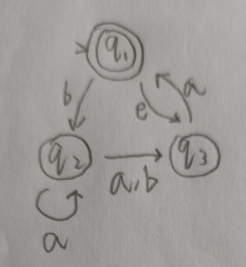t_states = ["q1", "q2", "q3"]
t_input = ["a", "b"]
t_trans = {
"q1":
{
"e": ["q3"],
"b": ["q2"]
},
"q2":
{
"a": ["q2", "q3"],
"b": ["q3"]
},
"q3":
{
"a": ["q1"],
},
}
t_start = "q1"
t_final = ["q1"]
nfa = NFA(t_states, t_input, t_trans, t_start, t_final)

输入可接受字符串baba，获得结果：
['q1'] -> ['q2']
['q2'] -> ['q2', 'q3']
['q2', 'q3'] -> ['q3']
['q3'] -> ['q1', 'q3']
被接受

输入不可接受字符串babaab，获得结果：
['q1'] -> ['q2']
['q2'] -> ['q2', 'q3']
['q2', 'q3'] -> ['q3']
['q3'] -> ['q1', 'q3']
['q1', 'q3'] -> ['q1', 'q3']
['q1', 'q3'] -> ['q2']
不被接受

DFA判断字符串是否被接受
实现在DFA.py中
代码分析
def read_input(self, input_str):
# 遍历输入字符串字符，进行状态转移，若最终状态为终结状态，输出接收，否则输出错误
current_state = self.start_state
# 确认字符可靠性
for c in input_str:
if c not in self.input_symbols:
print("输入字符串包括非法字符")
return False
# 遍历
for c in input_str:
current_state = self._get_next(current_state, c)
if current_state in self.final_states:
print("被接受")
return True
else:
print("不被接受")
return False

def _get_next(self, current_state, input_char):
# current_state为当前状态，input_char为读入字符
current_trans = self.trans[current_state]
next_state = current_trans[input_char]
print("{}-{}-{}".format(current_state, input_char, next_state))
return next_state

最简单的功能。 首先遍历字符串，确定有没有字母表以外的字符。每次读入一个字符，找到其对应的转移规则字典，确定输入的字符key，获得转移后的状态作为当前状态，输出状态转移过程。直到读完每一个字符。 最终判断状态是否为终结状态，输出判断。
示例分析
输入如图所示的DFA。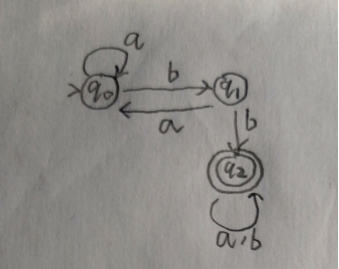t_start = "q0"
t_final = ['q2']
t_states = ["q0", "q1", "q2"]
t_symbols = ['a', 'b']
t_trans = {
'q0':
{
'a': 'q0',
'b': 'q1'
},
'q1':
{
'a': 'q0',
'b': 'q2'
},
'q2':
{
'a': 'q2',
'b': 'q2'
},
}
t_dfa = DFA.DFA(t_states, t_symbols, t_trans, t_start, t_final)

输入可接受字符串aaababb，获得结果：
q0-a-q0
q0-a-q0
q0-a-q0
q0-b-q1
q1-a-q0
q0-b-q1
q1-b-q2
被接受

输入不可接受字符串aba，获得结果：
q0-a-q0
q0-b-q1
q1-a-q0
不被接受

NFA转DFA
用的是表格法，计算所有状态组合以及组合能达到的闭包，最后生成DFA。
代码分析
计算表头
def create_table_head(states):
table_state_list = []
# 计算表头
state_num = len(states)
for i in range(state_num):
head_list = list(itertools.combinations(states, i + 1))
return table_state_list

首先计算转换表的表头，即状态集合的所有非空真子集。代码中利用itertools库的combinations方法获取组合结果的集合。
计算空闭包
		def cal_single_closure(s, trans):
# 计算单一状态闭包
closure = []
middle_state = deque()
closure.append(s)
middle_state.append(s)
while len(middle_state) > 0:
if middle_state not in trans.keys():
# 非确定可能不是所有状态都有转换规则
middle_state.popleft()
break
if dict(trans[middle_state]).keys().__contains__("e"):
next_states = dict(trans[middle_state])["e"]
for next_state in next_states:
if next_state not in closure:
closure.append(next_state)
middle_state.append(next_state)
middle_state.popleft()
return closure

输入为对应状态和NFA的转移规则。状态的空闭包为对应状态加上空规则的终点状态集合。代码创建一个队列middle_state，队列首先入队输入状态s，之后找到以s为起点的空转移规则，将结果放入队列，同时队列第一个状态出队列。以此循环，当middle_state为空时结束计算，返回闭包。
计算表格
def cal_table(input_symbols, table_state_num, table_state_list, trans, state_list, closures):
# table大小为表头*字母表长度,方便起见把所有项目都算上
table = []
for i in range(table_state_num):
simple_list =  * len(input_symbols)
# 空集合直接放入空结果
if 'blank' in table_state_list[i]:
for j in range(len(input_symbols)):
simple_list[j] = ('blank', )
table.append(simple_list)
continue
# 每个项目为转移规则结果的闭包的并集
# 获得转移结果
for j in range(len(input_symbols)):
simple_trans_result = []
for c in table_state_list[i]:
if c in trans.keys():
if input_symbols[j] in dict(trans)[c].keys():
next_states = dict(trans)[c][input_symbols[j]]
for next_state in next_states:
if next_state not in simple_trans_result:
simple_trans_result.append(next_state)
# 计算闭包并集
simple_closures_list = []
for k in simple_trans_result:
for c in closures[state_list.index(k)]:
if c not in simple_closures_list:
simple_closures_list.append(c)

# 直接利用顺序tuple进行对应
simple_closures_list = sorted(simple_closures_list)
if len(simple_closures_list) > 0:
simple_list[j] = tuple(simple_closures_list)
else:
simple_list[j] = tuple(["blank"])
table.append(simple_list)
return table

表格中的每个值，为表头中的状态接受对应字符后的转移结果的空闭包集合。存放结果为一个元组，包括所有闭包中的状态。空集合表头为(blank，)，对应的表项也都为(blank，)。 这步理解了基本就差不多了。
转换状态形式，生成转移规则
def trans_table(table, table_head):
# 为了计算方便，将状态combine转换为对应的index
index_table = copy.deepcopy(table)
for i in range(len(index_table)):
for k in range(len(index_table[i])):
combine = index_table[i][k]
index_table[i][k] = c_index
return index_table

def create_dfa_trans(table, start_index, symbols_list):
# 利用table构造dfa转换结果
trans = {}
states = []
middle_state = deque()
middle_state.append(start_index)
while len(middle_state) > 0:  # 注意还要加上对e兜底的判断
current_state = middle_state
states.append(current_state)
simple_rule = {}
for i in range(len(symbols_list)):
# 状态名用q+index表示
simple_rule[symbols_list[i]] = "q"+str(table[current_state][i])
if table[current_state][i] not in states:
middle_state.append(table[current_state][i])
trans["q"+str(current_state)] = simple_rule
middle_state.popleft()
return trans, states

将表格中的元组进行匹配，根据其在表头集合中的序号转换为新状态，即为q+序号的字符串。之后由开始状态创建对应的转移集合，利用队列进行所有状态的获取，直到队列为空。
获取终结状态
			def get_dfa_final_state(combine_list, final_states):
# 将combine_list中包含原终结状态的作为终结状态
final_combines = []
final_index = []
for c in combine_list:
for f in final_states:
if f in c:
final_combines.append(c)
break
for fc in final_combines:
f_index = get_combine_state_index(combine_list, fc)
final_index.append(f_index)
return final_index

终结状态为获取组合状态中有NFA中终结状态的组合状态。
最终将计算结果为参数创建DFA，返回即可。代码循环用的太多,并且表格其实不需要全部都算，算相关的即可，所以复杂度方面还是有点问题，优化啥的有空再说吧( ・_ゝ・)。
示例分析
将图中所示的NFA转换为DFA。def NFA_to_DFA():
t_start = "q1"
t_final = ['q1']
t_states = ["q1", "q2", "q3"]
t_symbols = ['a', 'b']
t_trans = {
'q1':
{
'b': ['q2'],
'e': ['q3']
},
'q2':
{
'a': ['q2', 'q3'],
'b': ['q3']
},
'q3':
{
'a': ['q1'],
},
}
t_nfa = NFA.NFA(t_states, t_symbols, t_trans, t_start, t_final)
t_dfa = DFA.trans_NFA(t_nfa)
print("start state:", end="")
print(t_dfa.start_state)
print("final states:", end="")
print(t_dfa.final_states)
print("states:", end="")
print(t_dfa.states)
print("trans:", end="")
print(t_dfa.trans)

输出中间表格结果：
a  b
blank   [('blank',), ('blank',)]
q1   [('blank',), ('q2',)]
q2   [('q2', 'q3'), ('q3',)]
q3   [('q1', 'q3'), ('blank',)]
q1,q2   [('q2', 'q3'), ('q2', 'q3')]
q1,q3   [('q1', 'q3'), ('q2',)]
q2,q3   [('q1', 'q2', 'q3'), ('q3',)]
q1,q2,q3   [('q1', 'q2', 'q3'), ('q2', 'q3')]

转换结果：
start state:q5
final states:[ 'q5', 'q7']
states:['q5', 'q2', 'q6', 'q3', 'q7', 'q3', 'q0']
trans:{'q5': {'a': 'q5', 'b': 'q2'}, 'q2': {'a': 'q6', 'b': 'q3'}, 'q6': {'a': 'q7', 'b': 'q3'}, 'q3': {'a': 'q5', 'b': 'q0'}, 'q7': {'a': 'q7', 'b': 'q6'}, 'q0': {'a': 'q0', 'b': 'q0'}}

转换后DFA示意图: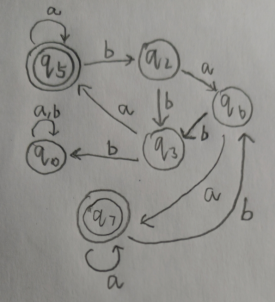DFA最小化
用的是划分法，思路就是找出等价状态。
代码分析
消去不可达状态
def _delete_unreachable_node(self):
# 删除不可达节点
# 所以先求可达节点
reachable_node = [self.start_state]
middle_state = deque()
middle_state.append(self.start_state)
while len(middle_state) > 0:
current_state = middle_state
for s in self.input_symbols:
state = self.trans[current_state][s]
if state not in reachable_node:
reachable_node.append(state)
middle_state.append(state)
middle_state.popleft()
# 删除不可达与对应转换规则
unreachable_node = []
for s in self.states:
if s not in reachable_node:
unreachable_node.append(s)
for un in unreachable_node:
self.states.remove(un)
del self.trans[un]

利用队列，从起始状态开始，将对应的所有转移结果状态中还未被标为可达状态的状态存入队列，同时消去队列第一个状态并归为可达状态，之后，利用可达状态求出不可达状态，并消去对应的状态和规则信息。

初始化划分信息
first = self.final_states.copy()
second = []
for s in self.states:
if s not in first:
second.append(s)
divide = [first, second]

初始划分为终结状态与其他状态。
计算新的划分信息
def _cal_divide(self, divide):
# 计算分离相关矩阵
whole_new_divide = []
for d in divide:
# 把归属都放在矩阵里
d_end = []
for char in self.input_symbols:
simple_d_end = []
for s in d:
end = self.trans[s][char]
# 找到end归哪组
for i in range(len(divide)):
if end in divide[i]:
simple_d_end.append(i)
break
d_end.append(simple_d_end)
# 展开新divide
new_divide = []
symbol_num = len(self.input_symbols)
for i in range(len(d_end)):
# 先把第一个放进去,先放序号
if len(new_divide) == 0:
new_divide.append([i])
else:
# 遍历已有新分组，放进去，不然就整个新的
is_in_old = False
for n_d in new_divide:
is_this = True
for j in range(symbol_num):
if d_end[j][n_d] != d_end[j][i]:
is_this = False
break
# 为true，分为该类
if is_this:
n_d.append(i)
is_in_old = True
break
# 整个新的
if not is_in_old:
new_divide.append([i])
# 此时新divide中全是index，变为对应状态
for n_d in new_divide:
for n_d_d_index in range(len(n_d)):
n_d[n_d_d_index] = d[n_d[n_d_d_index]]
for n_d in new_divide:
whole_new_divide.append(n_d)
return whole_new_divide

对每个划分进行判断。对d集合中的每个状态进行计算，生成矩阵。矩阵中信息为每个状态经过符号转移后状态所属的划分序号。只有所有符号的转移划分结果序号都分别相同时，两个状态才被分入同一个新的划分。
计算新转移
def _build_trans(self, divide):
# 利用新状态建成新转换
trans = {}
for i in range(len(divide)):
# 因为等价，所以用第一个状态即可
simple_rule = {}
state = divide[i]
for s in self.input_symbols:
end_state = self.trans[state][s]
after_end_state = ""
for j in range(len(divide)):
if end_state in divide[j]:
after_end_state = "q" + str(j)
break
simple_rule[s] = after_end_state
after_state = "q" + str(i)
trans[after_state] = simple_rule
return trans

每个划分给予一个状态，即为q+划分序号。同时依照每个划分中原有的转移信息生成新的转移规则。
示例分析
将图中所示DFA最小化。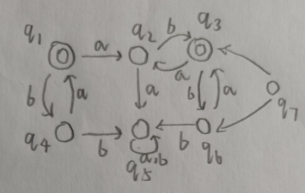划分结果：
	[['q1', 'q3'], ['q2'], ['q4', 'q6'], ['q5']]

新DFA结果五元组：
q0
['q0']
['q0', 'q1', 'q2', 'q3']
{'q0': {'a': 'q1', 'b': 'q2'}, 'q1': {'a': 'q3', 'b': 'q0'}, 'q2': {'a': 'q0', 'b': 'q3'}, 'q3': {'a': 'q3', 'b': 'q3'}}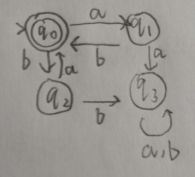RE与DFA转换
RE就是正则表达式，正则语言和FA之间关系紧密。 不过我转换只写了DFA转RE，RE转DFA要先逆波兰转换然后各个成分转规则，过于复杂，有兴趣自己实现下吧。
代码分析
增加伪开始状态与伪结束状态
trans = copy.deepcopy(self.trans)
# 增加假头和假尾
start_state = self.start_state
trans["qs"] = {"e": start_state}
for f_s in self.final_states:
trans[f_s]["e"] = "qe"

对开始状态增加到达它的一条空转移，对所有的终结状态增加从它出发的空转移。
获取状态上目标状态为自己的转移，转为∪连接的字符串 这步比较关键，主要是找到最终RE中被星号循环包裹的一部分。
def _make_self_rule_into_one(state, state_trans):
self_str = ""
self_rule_list = []
for t in dict(state_trans).keys():
# 自交为并
if state_trans[t] == state:
self_rule_list.append(t)
del state_trans[t]
# 数量大于1，则增加并符
if len(self_rule_list) == 1:
# state_trans[self_rule_list] = state
self_str = self_rule_list
elif len(self_rule_list) > 1:
self_to_self = "∪".join(self_rule_list)
# state_trans[self_to_self] = state
self_str = self_to_self
# 返回对应要放到星号中的符号串
return self_str

遍历对应状态上所有转移规则，若转移规则的终状态为本身，则将该转移规则的接受字符保存在list中，完成后将list中的字符串利用∪符号连接。
消去状态，产生新规则
# 依次消除状态
states = self.states.copy()
for state in states:
# 先把自交的归成一个
state_trans = trans[state]  # 当前状态为头
self_str = self._make_self_rule_into_one(state, state_trans)
# 计算每一条到达的规则和所有离开的规则的笛卡尔积
for k in trans.keys():
out_list = []
del_q = []
for q in dict(trans[k]).keys():
if trans[k][q] == state:
del_q.append(q)
# 找到所有出去的规则, (起点，入符号，出符号，终点)
for out_q in trans[state].keys():
out_list.append((k, q, out_q, trans[state][out_q]))
# 为了不破坏key，先删除，后添加
for q in del_q:
del trans[k][q]
for ol in out_list:
if len(self_str) > 1:
trans[k][ol + "({})*".format(self_str) + ol] = ol
elif len(self_str) == 1:
trans[k][ol + "{}*".format(self_str) + ol] = ol
# 删除自身规则
del trans[state]

将每条连向该状态的转移，和所有从该状态出的转移结合，将其对应的字符串连接，同时中间加上上一步算出的自己到达自己的中间字符串结果。最后添加新规则。
示例分析
将图中DFA转换为RE。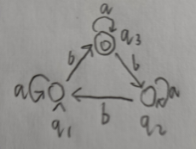def DFA_to_RL():
t_start = "q1"
t_final = ["q3"]
t_states = ["q1", "q2", "q3"]
t_symbols = ['a', 'b']
t_trans = {
"q1": {
"a": "q1",
"b": "q3"
},
"q2": {
"a": "q2",
"b": "q1"
},
"q3": {
"a": "q3",
"b": "q2"
},
}
t_dfa = DFA.DFA(t_states, t_symbols, t_trans, t_start, t_final)
t_rl = t_dfa.trans_to_RL()
print(t_rl)

输出结果： a*b(a∪ba*ba*b)*
总结
本项目主要实现了DFA与NFA相应的一些算法，以及与RE正则的转换，相对来说还算完整，有学编译原理或者计算理论整不明白的可以参考看看。
展开全文算法 python
• 确定有穷自动机就是说当一个状态面对一个输入符号的时候，它所转换到的是一个唯一确定的状态。 表示 例子 那么可以根据上面的5元组，绘制出下面的状态转移图。 解释：q0,q1,q2都是状态，所以都是节点，其中q0是...
DFA:deterministic finite automator
介绍
概念：

有穷自动机的每一步操作都是确定的，因此可称为确定型有穷自动机。确定有穷自动机就是说当一个状态面对一个输入符号的时候，它所转换到的是一个唯一确定的状态。

表示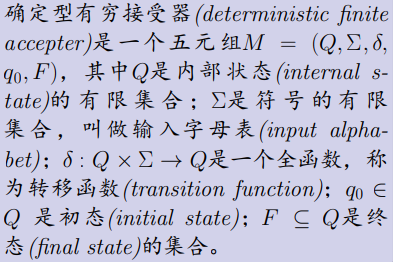例子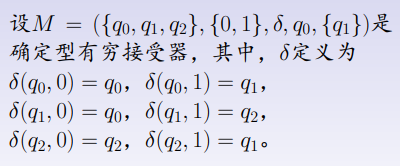那么可以根据上面的5元组，绘制出下面的状态转移图。 解释：q0,q1,q2都是状态，所以都是节点，其中q0是开始状态，q1是终止状态（我打了两个圈）。{0，1}表示输入串中允许出现的符号，比如给你的可能是001010等等。而

δ

(

q

0

,

0

)

=

q

0

\delta(q_0,0)=q_0

表示当你当前处于状态q0时，如果输入串给了你一个输入符号0，那么请你转移到状态q0。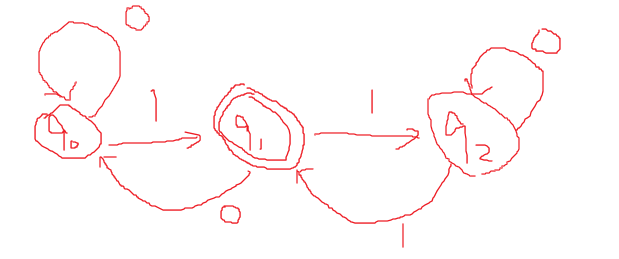我们试一下这个DFA是否可以接受这样的符号串0111。我们按照转移函数一个个走就行了，注意初始状态是q0,符号串的第一个符号是0。 即： 当前状态：q0 当前输入符号：0 剩余符号：111 所以：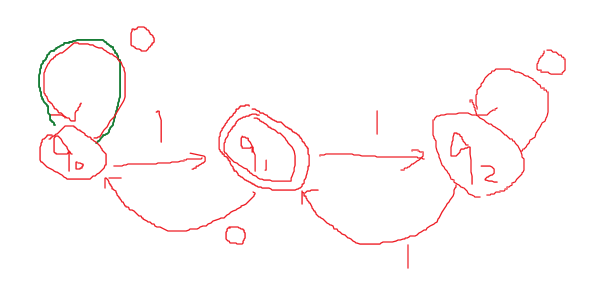即： 当前状态：q0 当前输入符号：1 剩余符号：11 接下来依次类推，最终有：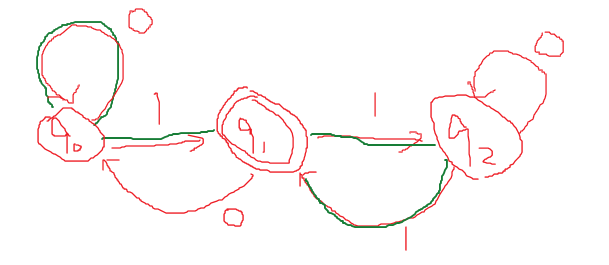从而最终进入了终态，q1。也就是说0111将被这个DFA接受，但是如果你提供了一个字符串0010，你会发现DFA不会接受。
化简
为什么需要化简？ 例子： 化简类型1，删除无用状态，例如下面的q2是不可能从初始状态q0到达的，所以没有用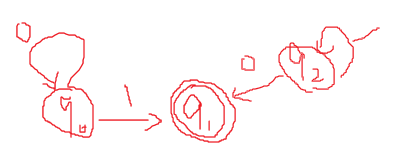化简类型2 注：q0是开始，q1是结束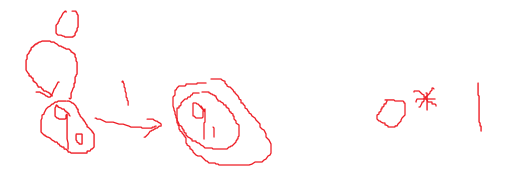容易看出来这个DFA是接受形如01的符号串，0表示0可以出现任意多次，包括0次。 我们再看下一个;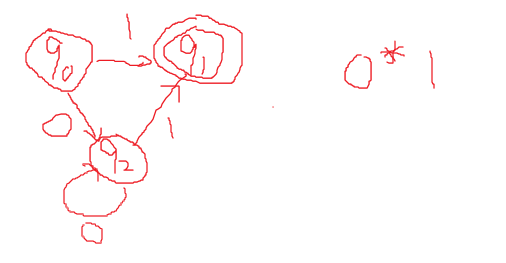我们发现，上面这个DFA也是接受0*1，也就是说这两个DFA功能一样，接受一样的语言，但是定义却不一样，我们希望找到一个方法，来选择简单的那个DFA,比如这里的第一个DFA，其状态更少，更加简洁。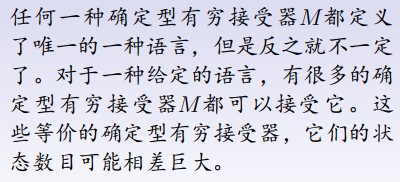如何化简？ 分割法： 为了简单起见，我们以上面的DFA2为例，大概走一个流程即好。 步骤1，对所有状态进行切分，先划分为两个集合，这样划分的原因是，这两种类型的状态一定是不一样的，也就是一定是可以区分的：
I0 = {q0,q2} ; 非终态

I1 = {q1} ; 终态


步骤2，对I0,I1状态继续进行切分，至于能不能继续切分我们不知道，但是要试一下，I1就不用切分了，因为里面只有一个状态，不可能可以分成两个集合。 但是I0里面有两个状态，可能是可区分的，我们得试一试，如何试一试？方法如下：
move(q0,0) = q2 ∈I0

move(q0,1) = q1 ∈I1

move(q2,0) = q2 ∈I0

move(q2,1) = q1 ∈I1

可以发现，q0,q1是等价的.


解释，上面的move就是那个转移函数

δ

\delta

。然后，我们还发现q0，q1读入一个0都转移到了I0（注意，现在我们的视角要上升到我们之前划分的集合I0,I1了。）q0,q1读入一个1都转移到了I1，所以q0,q1完全相同，不需要再进行拆分！也就是说最终的状态集合变成了I0,I1。 那么根据DFA的定义，我们还需要转移函数啊？哪来？ 注意到I0里面的任意元素都是等价的，所以你可以随便找上面的转移函数拿来用，比如根据上面的move有:
move(q0,0) = I0

move(q0,1) = I1

改成：
move(I0,0) = I0

move(I0,1) = I1

从而最终就是：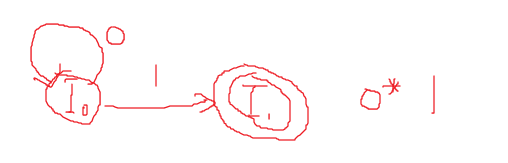至此我们的化简就结束了。
例子2
假设有如下DFA，试着将其最小化。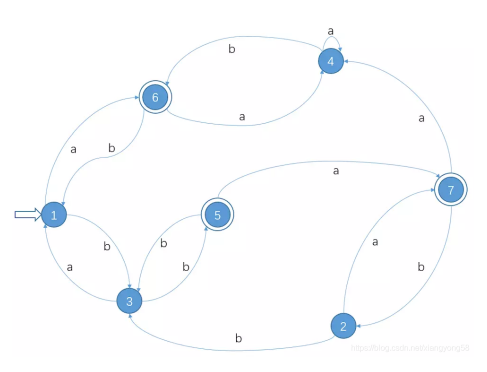分割成I0，I1
I0 = {1,2,3,4} ; 非终态

I1 = {5,6,7} ; 终态


检验I0中元素的等价性，不等价就分割
move(1,a) = 6 ∈I1

move(1,b) = 3 ∈I0

move(2,a) = 7 ∈I1

move(2,b) = 3 ∈I0

move(3,a) = 1 ∈I0

move(3,b) = 5 ∈I1

move(4,a) = 4 ∈I0

move(4,b) = 6 ∈I1

可以发现，{1,2}是等价的,{3,4}是等价的


所以现在分割成了：I2 = {1,2}, I1 = {5,6,7}, I3 = {3,4}，注意I0已经不复存在了，现在只有这3个状态集合。 检测I2中元素的等价性，不等价就分割
move(1,a) = 6 ∈I1

move(1,b) = 3 ∈I3

move(2,a) = 7 ∈I1

move(2,b) = 3 ∈I3

可以发现，是等价的，不用分割


检测I3中元素的等价性，不等价就分割
move(3,a) = 1 ∈I2

move(3,b) = 5 ∈I1

move(4,a) = 4 ∈I3

move(4,b) = 6 ∈I1
可以发现，不是等价的，分割成{3},{4}


所以现在分割成了：I2 = {1,2}, I1 = {5,6,7}, I4 = {3}, I5 = {4} 检测I1中元素的等价性，不等价就分割
move(5,a)  = 7 ∈ I1
move(5,b)  = 3 ∈ I4

move(6,a)  = 4 ∈ I5
move(6,b)  = 1 ∈ I2

move(7,a)  = 4 ∈ I5
move(7,b)  = 2 ∈ I2

可以发现，不是等价的，分割成{6,7}, {5}


所以现在分割成了：I2 = {1,2}, I4 = {3}, I5 = {4}, I6 = {5},I7 = {6,7}
检测后发现，不可再分割，所以最终分割结果就是：I2 = {1,2}, I4 = {3}, I5 = {4}, I6 = {5},I7 = {6,7} 最终结果为：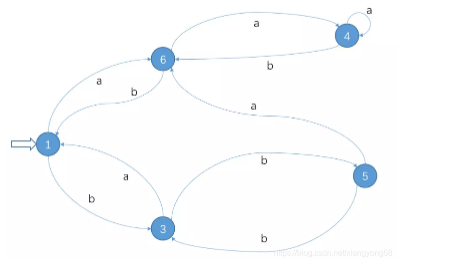注意顶点的名字随便取。上面是1,3,4,5,6完全也可以是其他的不同符号，予以区别就行。
应用
DFA的应用是和正则文法的等价性，和正则表达式也是等价的，所以可能用来分析一个给定的输入串是否合法，可以用于编译前端的词法分析：比如分析一个变量的名字是否为合法的标识符，假设有一种编程语言要求：变量命名必须开头是字母，后面是字母或者数字。那么可以有如下DFA来帮助我们识别用户的命名是否合法：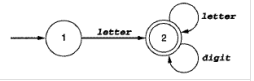其中letter表示字母，可以大小写，digit表示一个数字。
展开全文编译器
• 令s是一个代表状态，而且假设：在DFA M中，输入为a时从s到t转换。令t所在组的代表是r,那么在M’中有一个从s到r的转换，标记为a。令包含s0的状态组的代表是M’的开始状态，并令M’的接受状态是那些属于F的状态所在...
• 输入：非确定有穷状态自动机NFA 输出：确定化的有穷状态自动机DFA
• 1、确定有穷自动机DFA(Deterministic Finite Automata) M = ( S，Σ ，δ，s0，F )  S：有穷状态集  Σ：输入字母表，即输入符号集合。假设ε不是 Σ中的元素  δ：将S×Σ映射到S的转换函数。s∈S, a...
• DFA.java 中的DFA 类实现成员函数boolean recongnizeString(int move[][], int accept_state[], String word)
• 词法分析： 由正规式构造确定有穷自动机DFA 解题方法 1. 先由正规式构造转换系统 规则见下图： 2. 再由转换系统构造确定有穷自动机DFA (1) 求 Ia 假定 I 是转换图状态集 K 一个子集，Ia 是 I 中状态经历 一条 a...编译原理 词法分析 状态转换图 正规式
• 一个DFA可以通过消除无用状态、合并等价状态而转换成一个与之等价的最小状态的有穷自动机。 无用状态：从自动机开始状态出发，任何输入串也发到达的那个状态，或者这个状态没有通路可达终态。 等价转态：两个状态，...
• NFA和DFA都是有限状态机，一个是确定的，一个是确定的，这里的确定和不确定应该这么理解呢？ 其实确定和不确定指的是一个状态到另一个状态的输入是否是确定的。拿NFA和DFA的状态转换图来说就是：NFA同一个符号可以...
• DFA的每个状态都是一个由NFA中的状态构成的集合,即NFA状态集合的一个子集 r =aa*bb*cc* 二、从带ε-边的NFA到DFA的转换 r=0*1*2* 三、子集构造法（ subset construction）  输入：NFA N 输出...
• 一个五元组 M=(S,∑,f,S0,F) ...是单值部分映射，每个状态面临一个输入符号时，转入的后继状态是确定的。 S0∈S：唯一初态 F∈S：终态集(可空) 转载于:https://www.cnblogs.com/mznsndy/p/10712701.html...
• 对于P中的一个集合I，寻找I每一个元素K，找到K从边a对应的节点，加入集合I1，若I1是P中某个集合的子集，跳至步骤3，若不是，步骤4. 3，  寻找P中下一个集合，执行步骤2，若所有集合均是子集，则步骤5. 4，  将I1...算法 编译原理
• 使用Java模拟DFA 有穷自动机的执行过程。给出一个字符，判断是否能够被接受 使用Java模拟DFA 有穷自动机的执行过程。给出一个字符，判断是否能够被接受
• 1. 根据上面的状态转换...状态转换表是转台转换图的另外种表示形式，如下图，左侧表头0~9表示的 是状态，上方表头a,b,c表示的是条件。其余部分表示的是后继状态 a b ε 0∅∅1, 71∅∅2, 423∅∅3∅∅64∅5∅5∅...
• 1.1词法分析器生成工具Lex 虽然在学习上，我们学习的是Lex，但是最近经常使用的是词法分析器生成工具是Flex，它可以为C语言生成代码...在Flex中，它支持使用正则表达式来描述各个词法单元的模式，由此给出一个词...词法分析
• 确定有穷自动机(DFA)代码实现 代码思路： 采用类似于邻接矩阵的二维数组M存储上述的图结构。首先定义转换函数: $T:S_i×c → S_j, c∈\Sigma$ 该矩阵的行索引表示结点SiS_iSi​,列索引为对应的字母ccc,ccc为字母...词法分析 编译原理
• ## 编译原理：有穷自动机（DFA与NFA）

万次阅读 多人点赞 2018-03-01 19:38:25
我的机器学习教程「美团」算法工程师带你入门机器学习 已经开始更新了，欢迎大家订阅~ 任何关于算法、编程、AI行业知识或博客内容的问题，可以随时扫码关注公众号「图灵的猫」，加入”学习小组“，沙雕博主在线答疑...编译原理 离散数学 编程 算法
• dfa确定有限自动机定义In Deterministic Finite Automata (DFA), for a given input symbol the machine will move to which next state can be determined and hence it is named as deterministic automata....java python linux 数据库 算法
• 文章目录敏感词过滤 - DFA算法[确定有穷自动机]的Java 实现 敏感词过滤 - DFA算法[确定有穷自动机]的Java 实现 代码如下 package utils; import com.google.common.collect.Maps; import java.util.*; /** * @...敏感词过滤 Java实现python
• 分类（2）确定有穷自动机DFA）1.定义2.例子(重要！)2.2.状态转换图:2.3.状态转换矩阵3.DFA识别（接受字符串）①例子：（3）不确定有穷自动机（NFA）1.定义2.例子（重要！）:3.NFA识别的字符串（看一下就好了，跟...
• 形式语言与自动机恰好这样的题目，给出可输入变量的集合，然后构造一个可接受某种语言的自动机，对于这道题的要求就相当于对可接受语言的描述，而给出的字符串就是随机给出的语言，我们只需要构造好对应语言的DFA...算法 字符串 数据结构
• php实现基于确定有穷自动机算法的铭感词过滤 安装&使用流程 Download and install Composer: curl -sS https://getcomposer.org/installer | php 要检查 Composer 是否正常工作，只需要通过 php 来执行 PHAR ...
• ## 确定有穷自动机

千次阅读 2017-04-08 11:35:26
确定有穷自动机 思路: 将状态与对应的行，符号与对应的列 索引进行映射,输入规则为状态转换表,之后输入待判断的字符串,判断是否会被自动机接受 package com.parting_soul; import java.util.HashMap; ...编译原理...